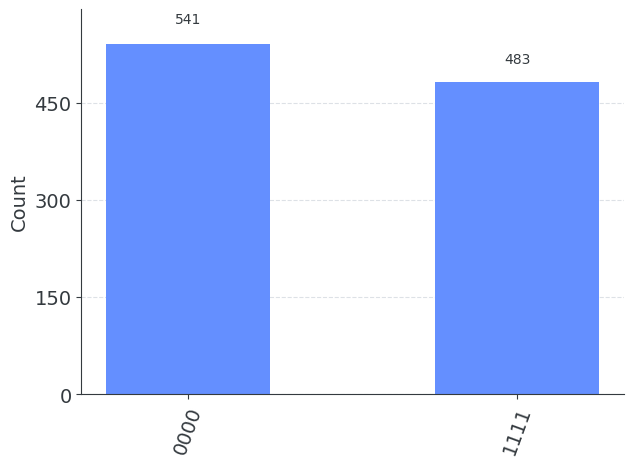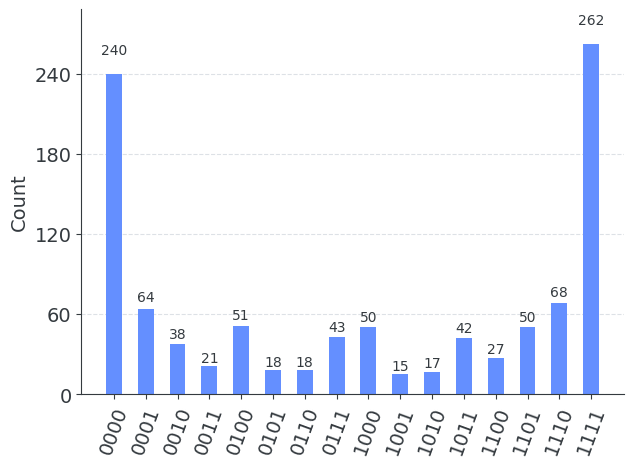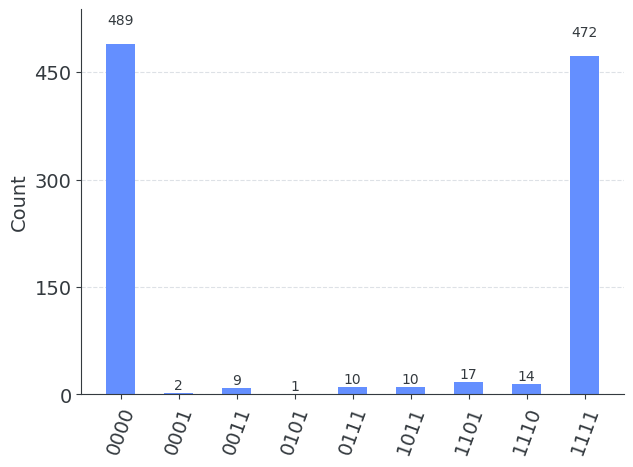# Building noise models

This page shows how to use the Qiskit Aer noise (opens in a new tab) module to build noise models for simulating quantum circuits in the presence of errors. This is useful for emulating noisy quantum processors and for studying the effects of noise on the execution of quantum algorithms.

 :
import numpy as np
from qiskit import QuantumCircuit
from qiskit.quantum_info import Kraus, SuperOp
from qiskit.tools.visualization import plot_histogram
from qiskit.transpiler.preset_passmanagers import generate_preset_pass_manager
from qiskit_aer import AerSimulator

# Import from Qiskit Aer noise module
from qiskit_aer.noise import (
NoiseModel,
QuantumError,
depolarizing_error,
pauli_error,
thermal_relaxation_error,
)

## Qiskit Aer noise module

The Qiskit Aer noise module contains Python classes to build customized noise models for simulation. There are three key classes:

1. The NoiseModel class which stores a noise model used for noisy simulation.

2. The QuantumError class which describes CPTP gate errors. These can be applied:

• After gate or reset instructions
• Before measure instructions.
3. The ReadoutError class which describes classical readout errors.

## Initializing a noise model from a backend

You can initialize a noise model with parameters set from the latest calibration data for a physical backend:

 :
from qiskit_ibm_runtime import QiskitRuntimeService

service = QiskitRuntimeService()
backend = service.backend("ibm_brisbane")
noise_model = NoiseModel.from_backend(backend)

This will yield a noise model that roughly approximates the errors one would encounter when using that backend. If you want to have more detailed control over the parameters of the noise model, then you'll need to create your own noise model, as described in the rest of this page.

## Quantum Errors

Rather than deal with the QuantumError object directly, many helper functions exist to automatically generate a specific type of parameterized quantum error. These are contained in the noise module and include functions for many common errors types used in quantum computing research. The function names and the type of error they return are:

Standard error functionDetails
kraus_errora general n-qubit CPTP error channel given as a list of Kraus matrices $[K_0, ...]$.
mixed_unitary_erroran n-qubit mixed unitary error given as a list of unitary matrices and probabilities $[(U_0, p_0),...]$.
coherent_unitary_erroran n-qubit coherent unitary error given as a single unitary matrix $U$.
pauli_erroran n-qubit Pauli error channel (mixed unitary) given as a list of Pauli's and probabilities $[(P_0, p_0),...]$
depolarizing_erroran n-qubit depolarizing error channel parameterized by a depolarization probability $p$.
reset_errora single-qubit reset error parameterized by a probabilities $p_0, p_1$ of resetting to the \$
thermal_relaxation_errora single qubit thermal relaxation channel parameterized by relaxation time constants $T_1$, $T_2$, gate time $t$, and excited state thermal population $p_1$.
phase_amplitude_damping_errorA single-qubit generalized combined phase and amplitude damping error channel given by an amplitude damping parameter $\lambda$, a phase damping parameter $\gamma$, and an excited state thermal population $p_1$.
amplitude_damping_errorA single-qubit generalized amplitude damping error channel given by an amplitude damping parameter $\lambda$, and an excited state thermal population $p_1$.
phase_damping_errorA single-qubit phase damping error channel given by a phase damping parameter $\gamma$

### Combining quantum errors

QuantumError instances can be combined by using composition, tensor product, and tensor expansion (reversed order tensor product) to produce new QuantumErrors as:

• Composition: $\cal{E}(\rho)=\cal{E_2}(\cal{E_1}(\rho))$ as error = error1.compose(error2)
• Tensor product: $\cal{E}(\rho) =(\cal{E_1}\otimes\cal{E_2})(\rho)$ as error error1.tensor(error2)
• Expand product: $\cal{E}(\rho) =(\cal{E_2}\otimes\cal{E_1})(\rho)$ as error error1.expand(error2)

### Example

For example to construct a 5% single-qubit Bit-flip error:

 :
# Construct a 1-qubit bit-flip and phase-flip errors
p_error = 0.05
bit_flip = pauli_error([('X', p_error), ('I', 1 - p_error)])
phase_flip = pauli_error([('Z', p_error), ('I', 1 - p_error)])
print(bit_flip)
print(phase_flip)

Output:

QuantumError on 1 qubits. Noise circuits:
P(0) = 0.05, Circuit =
┌───┐
q: ┤ X ├
└───┘
P(1) = 0.95, Circuit =
┌───┐
q: ┤ I ├
└───┘
QuantumError on 1 qubits. Noise circuits:
P(0) = 0.05, Circuit =
┌───┐
q: ┤ Z ├
└───┘
P(1) = 0.95, Circuit =
┌───┐
q: ┤ I ├
└───┘

 :
# Compose two bit-flip and phase-flip errors
bitphase_flip = bit_flip.compose(phase_flip)
print(bitphase_flip)

Output:

QuantumError on 1 qubits. Noise circuits:
P(0) = 0.0025000000000000005, Circuit =
┌───┐┌───┐
q: ┤ X ├┤ Z ├
└───┘└───┘
P(1) = 0.0475, Circuit =
┌───┐┌───┐
q: ┤ X ├┤ I ├
└───┘└───┘
P(2) = 0.0475, Circuit =
┌───┐┌───┐
q: ┤ I ├┤ Z ├
└───┘└───┘
P(3) = 0.9025, Circuit =
┌───┐┌───┐
q: ┤ I ├┤ I ├
└───┘└───┘

 :
# Tensor product two bit-flip and phase-flip errors with
# bit-flip on qubit-0, phase-flip on qubit-1
error2 = phase_flip.tensor(bit_flip)
print(error2)

Output:

QuantumError on 2 qubits. Noise circuits:
P(0) = 0.0025000000000000005, Circuit =
┌───┐
q_0: ┤ X ├
├───┤
q_1: ┤ Z ├
└───┘
P(1) = 0.0475, Circuit =
┌───┐
q_0: ┤ I ├
├───┤
q_1: ┤ Z ├
└───┘
P(2) = 0.0475, Circuit =
┌───┐
q_0: ┤ X ├
├───┤
q_1: ┤ I ├
└───┘
P(3) = 0.9025, Circuit =
┌───┐
q_0: ┤ I ├
├───┤
q_1: ┤ I ├
└───┘


### Converting to and from QuantumChannel operators

We can also convert back and forth between QuantumError objects in Qiskit Aer and QuantumChannel objects in Qiskit Terra.

 :
# Convert to Kraus operator
bit_flip_kraus = Kraus(bit_flip)
print(bit_flip_kraus)

Output:

Kraus([[[ 9.74679434e-01+0.j,  0.00000000e+00+0.j],
[-1.20234617e-16+0.j,  9.74679434e-01+0.j]],

[[ 2.62045272e-16+0.j,  2.23606798e-01+0.j],
[ 2.23606798e-01+0.j, -2.84112242e-16+0.j]]],
input_dims=(2,), output_dims=(2,))

 :
# Convert to Superoperator
phase_flip_sop = SuperOp(phase_flip)
print(phase_flip_sop)

Output:

SuperOp([[1. +0.j, 0. +0.j, 0. +0.j, 0. +0.j],
[0. +0.j, 0.9+0.j, 0. +0.j, 0. +0.j],
[0. +0.j, 0. +0.j, 0.9+0.j, 0. +0.j],
[0. +0.j, 0. +0.j, 0. +0.j, 1. +0.j]],
input_dims=(2,), output_dims=(2,))

 :
# Convert back to a quantum error
print(QuantumError(bit_flip_kraus))

# Check conversion is equivalent to original error
QuantumError(bit_flip_kraus) == bit_flip

Output:

QuantumError on 1 qubits. Noise circuits:
P(0) = 1.0, Circuit =
┌───────┐
q: ┤ kraus ├
└───────┘

True


Classical readout errors are specified by a list of assignment probabilities vectors $P(A|B)$:

• $A$ is the recorded classical bit value
• $B$ is the true bit value returned from the measurement

E.g. for 1 qubits: $P(A|B) = [P(A|0), P(A|1)]$.

 :
# Measurement miss-assignement probabilities
p0given1 = 0.1
p1given0 = 0.05

ReadoutError([[1 - p1given0, p1given0], [p0given1, 1 - p0given1]])

Output:

ReadoutError([[0.95 0.05]
[0.1  0.9 ]])


Readout errors may also be combined using compose, tensor and expand like with quantum errors.

## Adding errors to a Noise Model

When adding a quantum error to a noise model we must specify the type of instruction that it acts on, and what qubits to apply it to. There are two cases for Quantum Errors:

1. All-qubit quantum error
2. Specific qubit quantum error

### 1. All-qubit quantum error

This applies the same error to any occurrence of an instruction, regardless of which qubits it acts on.

It is added as noise_model.add_all_qubit_quantum_error(error, instructions):

 :
# Create an empty noise model
noise_model = NoiseModel()

# Add depolarizing error to all single qubit u1, u2, u3 gates
error = depolarizing_error(0.05, 1)

# Print noise model info
print(noise_model)

Output:

NoiseModel:
Basis gates: ['cx', 'id', 'rz', 'sx', 'u1', 'u2', 'u3']
Instructions with noise: ['u1', 'u3', 'u2']
All-qubits errors: ['u1', 'u2', 'u3']


### 2. Specific qubit quantum error

This applies the error to any occurrence of an instruction acting on a specified list of qubits. Note that the order of the qubit matters: For a 2-qubit gate an error applied to qubits [0, 1] is different to one applied to qubits [1, 0] for example.

It is added as noise_model.add_quantum_error(error, instructions, qubits):

 :
# Create an empty noise model
noise_model = NoiseModel()

# Add depolarizing error to all single qubit u1, u2, u3 gates on qubit 0 only
error = depolarizing_error(0.05, 1)

# Print noise model info
print(noise_model)

Output:

NoiseModel:
Basis gates: ['cx', 'id', 'rz', 'sx', 'u1', 'u2', 'u3']
Instructions with noise: ['u1', 'u3', 'u2']
Qubits with noise: 
Specific qubit errors: [('u1', (0,)), ('u2', (0,)), ('u3', (0,))]


### Note on non-local qubit quantum error

NoiseModel does not support addition of non-local qubit quantum errors. They should be handled outside of NoiseModel. That suggests you should write your own transpiler pass (TransformationPass) and run the pass just before running the simulator if you need to insert your quantum errors into your circuit under your own conditions.

### Executing a noisy simulation with a noise model

The command AerSimulator(noise_model=noise_model) returns a simulator configured to the given noise model. In addition to setting the simulator's noise model, it also overrides the simulator's basis gates, according to the gates of the noise model.

## Noise Model Examples

We will now give some examples of noise models. For our demonstrations we will use a simple test circuit generating a n-qubit GHZ state:

 :
# System Specification
n_qubits = 4
circ = QuantumCircuit(n_qubits)

# Test Circuit
circ.h(0)
for qubit in range(n_qubits - 1):
circ.cx(qubit, qubit + 1)
circ.measure_all()
print(circ)

Output:

        ┌───┐                ░ ┌─┐
q_0: ┤ H ├──■─────────────░─┤M├─────────
└───┘┌─┴─┐           ░ └╥┘┌─┐
q_1: ─────┤ X ├──■────────░──╫─┤M├──────
└───┘┌─┴─┐      ░  ║ └╥┘┌─┐
q_2: ──────────┤ X ├──■───░──╫──╫─┤M├───
└───┘┌─┴─┐ ░  ║  ║ └╥┘┌─┐
q_3: ───────────────┤ X ├─░──╫──╫──╫─┤M├
└───┘ ░  ║  ║  ║ └╥┘
meas: 4/════════════════════════╩══╩══╩══╩═
0  1  2  3


### Ideal Simulation

 :
# Ideal simulator and execution
sim_ideal = AerSimulator()
result_ideal = sim_ideal.run(circ).result()
plot_histogram(result_ideal.get_counts(0))

Output:## Noise Example 1: Basic bit-flip error noise model

Lets consider a simple toy noise model example common in quantum information theory research:

• When applying a single qubit gate, flip the state of the qubit with probability p_gate1.
• When applying a 2-qubit gate apply single-qubit errors to each qubit.
• When resetting a qubit reset to 1 instead of 0 with probability p_reset.
• When measuring a qubit, flip the state of the qubit with probability p_meas.
 :
# Example error probabilities
p_reset = 0.03
p_meas = 0.1
p_gate1 = 0.05

# QuantumError objects
error_reset = pauli_error([('X', p_reset), ('I', 1 - p_reset)])
error_meas = pauli_error([('X',p_meas), ('I', 1 - p_meas)])
error_gate1 = pauli_error([('X',p_gate1), ('I', 1 - p_gate1)])
error_gate2 = error_gate1.tensor(error_gate1)

# Add errors to noise model
noise_bit_flip = NoiseModel()

print(noise_bit_flip)

Output:

NoiseModel:
Basis gates: ['cx', 'id', 'rz', 'sx', 'u1', 'u2', 'u3']
Instructions with noise: ['measure', 'u2', 'cx', 'u1', 'u3', 'reset']
All-qubits errors: ['reset', 'measure', 'u1', 'u2', 'u3', 'cx']


### Executing the noisy simulation

 :
# Create noisy simulator backend
sim_noise = AerSimulator(noise_model=noise_bit_flip)

# Transpile circuit for noisy basis gates
passmanager = generate_preset_pass_manager(optimization_level=3, backend=sim_noise)
circ_tnoise = passmanager.run(circ)

# Run and get counts
result_bit_flip = sim_noise.run(circ_tnoise).result()
counts_bit_flip = result_bit_flip.get_counts(0)

# Plot noisy output
plot_histogram(counts_bit_flip)

Output:## Example 2: T1/T2 thermal relaxation

Now consider a more realistic error model based on thermal relaxation with the qubit environment:

• Each qubit is parameterized by a thermal relaxation time constant $T_1$ and a dephasing time constant $T_2$.
• Note that we must have $T_2 \le 2 T_1$.
• Error rates on instructions are determined by gate times and qubit $T_1$, $T_2$ values.
 :
# T1 and T2 values for qubits 0-3
T1s = np.random.normal(50e3, 10e3, 4) # Sampled from normal distribution mean 50 microsec
T2s = np.random.normal(70e3, 10e3, 4)  # Sampled from normal distribution mean 50 microsec

# Truncate random T2s <= T1s
T2s = np.array([min(T2s[j], 2 * T1s[j]) for j in range(4)])

# Instruction times (in nanoseconds)
time_u1 = 0   # virtual gate
time_u2 = 50  # (single X90 pulse)
time_u3 = 100 # (two X90 pulses)
time_cx = 300
time_reset = 1000  # 1 microsecond
time_measure = 1000 # 1 microsecond

# QuantumError objects
errors_reset = [thermal_relaxation_error(t1, t2, time_reset)
for t1, t2 in zip(T1s, T2s)]
errors_measure = [thermal_relaxation_error(t1, t2, time_measure)
for t1, t2 in zip(T1s, T2s)]
errors_u1  = [thermal_relaxation_error(t1, t2, time_u1)
for t1, t2 in zip(T1s, T2s)]
errors_u2  = [thermal_relaxation_error(t1, t2, time_u2)
for t1, t2 in zip(T1s, T2s)]
errors_u3  = [thermal_relaxation_error(t1, t2, time_u3)
for t1, t2 in zip(T1s, T2s)]
errors_cx = [[thermal_relaxation_error(t1a, t2a, time_cx).expand(
thermal_relaxation_error(t1b, t2b, time_cx))
for t1a, t2a in zip(T1s, T2s)]
for t1b, t2b in zip(T1s, T2s)]

# Add errors to noise model
noise_thermal = NoiseModel()
for j in range(4):
for k in range(4):

print(noise_thermal)

Output:

NoiseModel:
Basis gates: ['cx', 'id', 'rz', 'sx', 'u2', 'u3']
Instructions with noise: ['measure', 'u2', 'cx', 'u3', 'reset']
Qubits with noise: [0, 1, 2, 3]
Specific qubit errors: [('reset', (0,)), ('reset', (1,)), ('reset', (2,)), ('reset', (3,)), ('measure', (0,)), ('measure', (1,)), ('measure', (2,)), ('measure', (3,)), ('u2', (0,)), ('u2', (1,)), ('u2', (2,)), ('u2', (3,)), ('u3', (0,)), ('u3', (1,)), ('u3', (2,)), ('u3', (3,)), ('cx', (0, 0)), ('cx', (0, 1)), ('cx', (0, 2)), ('cx', (0, 3)), ('cx', (1, 0)), ('cx', (1, 1)), ('cx', (1, 2)), ('cx', (1, 3)), ('cx', (2, 0)), ('cx', (2, 1)), ('cx', (2, 2)), ('cx', (2, 3)), ('cx', (3, 0)), ('cx', (3, 1)), ('cx', (3, 2)), ('cx', (3, 3))]


### Executing the noisy simulation

 :
# Run the noisy simulation
sim_thermal = AerSimulator(noise_model=noise_thermal)

# Transpile circuit for noisy basis gates
passmanager = generate_preset_pass_manager(optimization_level=3, backend=sim_thermal)
circ_tthermal = passmanager.run(circ)

# Run and get counts
result_thermal = sim_thermal.run(circ_tthermal).result()
counts_thermal = result_thermal.get_counts(0)

# Plot noisy output
plot_histogram(counts_thermal)

Output: